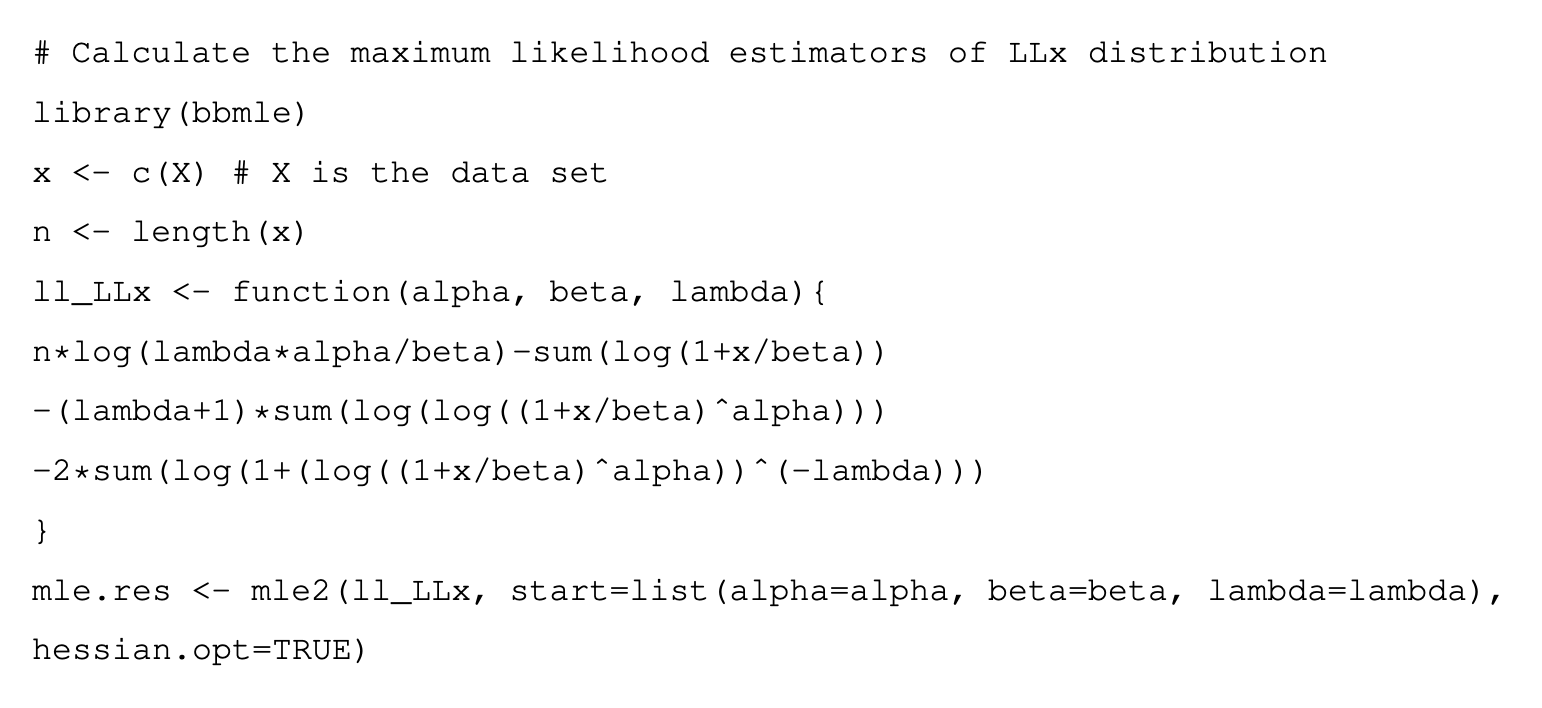# Pictures of code are not code

We’re in Covid lockdown again in Auckland, and it’s a weekend, so I happened to be on the Stats stackoverflow site.1 Someone was trying to use R to check MLE calculations for a few papers that propose new parametric models – the “overgeneralised Gamma distribution” genre of statistical literature. These paper typically have “real data sets”2, which might provide a good introduction to optimisation problems that are a bit harder than generalised linear models but don’t require any specialised knowledge.

The first example looks to be just wrong. The data were on the failure times of fifty “components”, not further specified; the distribution was a new modified half-logistic distribution with two extra shape parameters. The person asking the question worked out the MLE, and I duplicated his answer and also worked it out a different way. We got the same result, with higher loglikelihood than the estimate in the paper. I suspected a typo in the data, but it matches the textbook it was taken from. Who knows – or, really, cares?

The second paper actually gave R code, which is a big step forward. There were two problems with it, though. Here’s what the code looked likeI spent quite a while trying to get this to work. One potential issue I thought of was numerical instability, so I simplified the log(power) terms and used `log1p` to handle the `log(1+)` terms. Weirdly, the result didn’t change after some of my edits.

A function body in R consists of multiple `call` objects. These are evaluated in turn, and the value of the function is the value of the last call. The body of this function, specifically, consists of three `call` objects. The value of the function is the value of the last of the three: `-2 * sum(log(1 + (log((1 + x/beta)^alpha))^(-lambda)))`, just a fragment of the actual loglikelihood. You’d never have this problem with real code, but it’s easy with PDF or paper pictures of code. Either copy-pasting or retyping the code is likely to be wrong even without the potential for typos. PDF is not a code format; paper isn’t either. I’ve seen worse than this: a paper in JRSS A where the online appendix had code in a bitmap image.

Oh, the second problem? The code calls `mle2`, which expects to be given the negative loglikelihood, and so minimises it. The function `ll_LLx`, however, is the loglikelihood. So the code, if you fix the spurious line breaks to make it run as it putatively did for the authors, would give a minimum likelihood estimator. Fixing this second problem finally gives the answers in the paper, demonstrating that the authors did not get them from the code they provided.

1. I quite like the site; the ratio of people answering questions to people complaining about questions being bad is bearable and some of the questions are interesting↩︎

2. where “real data”, as Di Cook has pointed out, is a technical term meaning “not real data”↩︎